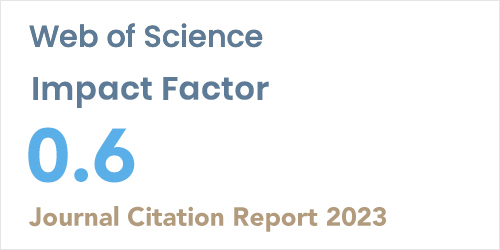ISSN: 1304-7191 | E-ISSN: 1304-7205
Volume: 41 Issue: 5 Year: 2023Vector autoregressive clustering for redundancy analysis in air pollution monitorıng networks at Türkiye
1Muğla Sıtkı Koçman University, Faculty of Science, Department of Statistics, Muğla, Türkiye
Sigma J Eng Nat Sci - DOI: 10.14744/sigma.2023.00074

#### Abstract

This study proposes a new approach to reduce the information redundancy at Air Pollution Monitoring Networks (APMNs) and costs required for monitoring them. Proposed approach is based on Vector Autoregressive (VAR) model which describes the relationship between multivariate time series and consists of three main steps: In the first step, VAR model between two or more than two time series consisting of air pollutant observations is estimated. This step is repeated as the number of monitoring stations (n) under study and thus, n parameter vectors are obtained. In the second step, parameters vectors are divided into homogenous groups by using clustering analysis. The objective of this step is to identify the similar monitoring stations in terms of the relationship. Last step is to calculate the reduced information redundancy and the monitoring costs. To evaluate the efficiency of proposed approach, data sets consisting of PM10 and SO2 time series obtained from 116 APMNs at Turkey are used. Fuzzy K-Medoids (FKM) as clustering method Xie-Beni (XB) index as cluster validity index are preferred. Experimental results showed that information redundancy and monitoring cost in PM10 and SO2 stations can reduced at the rate of 63.36 by following proposed approach.